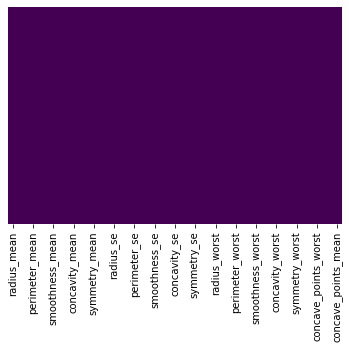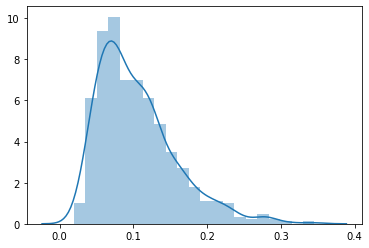# Feature Selection Techniques – Embedded Method (Lasso)

300320202027

Embedded methods are iterative in a sense that takes care of each iteration of the model training process and carefully extract those features which contribute the most to the training for a particular iteration. Regularization methods are the most commonly used embedded methods which penalize a feature given a coefficient threshold. Here we will do feature selection using Lasso regularization. If the feature is irrelevant, lasso penalizes its coefficient and make it 0. Hence the features with coefficient = 0 are removed and the rest are taken.

In :
import numpy as np
import pandas as pd
import seaborn as sns
import matplotlib.pyplot as plt
from sklearn.preprocessing import LabelEncoder, OneHotEncoder
import warnings
warnings.filterwarnings("ignore")
from sklearn.model_selection import train_test_split
from sklearn.svm import SVC
from sklearn.metrics import confusion_matrix
np.random.seed(123)

In :
##  colorful prints
def black(text):
print('33[30m', text, '33[0m', sep='')
def red(text):
print('33[31m', text, '33[0m', sep='')
def green(text):
print('33[32m', text, '33[0m', sep='')
def yellow(text):
print('33[33m', text, '33[0m', sep='')
def blue(text):
print('33[34m', text, '33[0m', sep='')
def magenta(text):
print('33[35m', text, '33[0m', sep='')
def cyan(text):
print('33[36m', text, '33[0m', sep='')
def gray(text):
print('33[90m', text, '33[0m', sep='')

In :
df = pd.read_csv ('/home/wojciech/Pulpit/6/Breast_Cancer_Wisconsin.csv')
green(df.shape)

(569, 33)

Out:
id diagnosis radius_mean texture_mean perimeter_mean area_mean smoothness_mean compactness_mean concavity_mean concave points_mean texture_worst perimeter_worst area_worst smoothness_worst compactness_worst concavity_worst concave points_worst symmetry_worst fractal_dimension_worst Unnamed: 32
0 842302 M 17.99 10.38 122.8 1001.0 0.11840 0.27760 0.3001 0.14710 17.33 184.6 2019.0 0.1622 0.6656 0.7119 0.2654 0.4601 0.11890 NaN
1 842517 M 20.57 17.77 132.9 1326.0 0.08474 0.07864 0.0869 0.07017 23.41 158.8 1956.0 0.1238 0.1866 0.2416 0.1860 0.2750 0.08902 NaN
2 84300903 M 19.69 21.25 130.0 1203.0 0.10960 0.15990 0.1974 0.12790 25.53 152.5 1709.0 0.1444 0.4245 0.4504 0.2430 0.3613 0.08758 NaN

3 rows × 33 columns

### Deleting unneeded columns¶

In :
df['concave_points_worst'] = df['concave points_worst']
df['concave_points_se'] = df['concave points_se']
df['concave_points_mean'] = df['concave points_mean']

del df['Unnamed: 32']
del df['diagnosis']
del df['id']

In :
df.isnull().sum()

Out:
radius_mean                0
texture_mean               0
perimeter_mean             0
area_mean                  0
smoothness_mean            0
compactness_mean           0
concavity_mean             0
concave points_mean        0
symmetry_mean              0
fractal_dimension_mean     0
texture_se                 0
perimeter_se               0
area_se                    0
smoothness_se              0
compactness_se             0
concavity_se               0
concave points_se          0
symmetry_se                0
fractal_dimension_se       0
texture_worst              0
perimeter_worst            0
area_worst                 0
smoothness_worst           0
compactness_worst          0
concavity_worst            0
concave points_worst       0
symmetry_worst             0
fractal_dimension_worst    0
concave_points_worst       0
concave_points_se          0
concave_points_mean        0
dtype: int64
In :
import seaborn as sns

sns.heatmap(df.isnull(),yticklabels=False,cbar=False,cmap='viridis')

Out:
<matplotlib.axes._subplots.AxesSubplot at 0x7f82c6742350>### Deletes duplicates¶

there were no duplicates

In :
green(df.shape)
df.drop_duplicates(keep='first', inplace=True)
blue(df.shape)

(569, 33)
(569, 33)

In :
blue(df.dtypes)

radius_mean                float64
texture_mean               float64
perimeter_mean             float64
area_mean                  float64
smoothness_mean            float64
compactness_mean           float64
concavity_mean             float64
concave points_mean        float64
symmetry_mean              float64
fractal_dimension_mean     float64
texture_se                 float64
perimeter_se               float64
area_se                    float64
smoothness_se              float64
compactness_se             float64
concavity_se               float64
concave points_se          float64
symmetry_se                float64
fractal_dimension_se       float64
texture_worst              float64
perimeter_worst            float64
area_worst                 float64
smoothness_worst           float64
compactness_worst          float64
concavity_worst            float64
concave points_worst       float64
symmetry_worst             float64
fractal_dimension_worst    float64
concave_points_worst       float64
concave_points_se          float64
concave_points_mean        float64
dtype: object

In :
df.columns

Out:
Index(['radius_mean', 'texture_mean', 'perimeter_mean', 'area_mean',
'smoothness_mean', 'compactness_mean', 'concavity_mean',
'concave points_mean', 'symmetry_mean', 'fractal_dimension_mean',
'compactness_se', 'concavity_se', 'concave points_se', 'symmetry_se',
'perimeter_worst', 'area_worst', 'smoothness_worst',
'compactness_worst', 'concavity_worst', 'concave points_worst',
'symmetry_worst', 'fractal_dimension_worst', 'concave_points_worst',
'concave_points_se', 'concave_points_mean'],
dtype='object')

### We choose the continuous variable – compactness_mean¶

In :
print('max:',df['compactness_mean'].max())
print('min:',df['compactness_mean'].min())

sns.distplot(np.array(df['compactness_mean']))

max: 0.3454
min: 0.01938

Out:
<matplotlib.axes._subplots.AxesSubplot at 0x7f82c66b0090># Lasso¶

In :
X = df.drop('compactness_mean', axis=1)
y = df['compactness_mean']


## I set the number of variables that will remain in the model¶

In :
Num_v = 15

In :
from sklearn import linear_model

#rlasso = RandomizedLasso(alpha=0.025)

# Standaryzacja zmiennych

clf = linear_model.Lasso(alpha=0.1, positive=True)
clf.fit(X, y)

blue(clf.coef_)
print()
green(clf.intercept_)
print()
red(clf.score(X,y))

[0.00000000e+00 0.00000000e+00 0.00000000e+00 0.00000000e+00
0.00000000e+00 0.00000000e+00 0.00000000e+00 0.00000000e+00
0.00000000e+00 0.00000000e+00 0.00000000e+00 0.00000000e+00
2.11821738e-05 0.00000000e+00 0.00000000e+00 0.00000000e+00
0.00000000e+00 0.00000000e+00 0.00000000e+00 0.00000000e+00
0.00000000e+00 8.17079026e-04 0.00000000e+00 0.00000000e+00
0.00000000e+00 0.00000000e+00 0.00000000e+00 0.00000000e+00
0.00000000e+00 0.00000000e+00 0.00000000e+00 0.00000000e+00]

0.015845670027763575

0.3452546166160324


The positive parameter, which on Truei forces the coefficients to be positive. In addition, setting alpha regularization to a value close to 0 (i.e., 0.001) causes Lasso to mimic linear regression without regularization.

### Metoda zip na wyświetlenie rankingu cech¶

In :
PPS = clf.coef_

KOT_lasso = dict(zip(df, PPS))
KOT_sorted_keys_lasso = sorted(KOT_lasso, key=KOT_lasso.get, reverse=True)

for r in KOT_sorted_keys_lasso:
print (r, (KOT_lasso[r]))

texture_worst 0.0008170790257354554
perimeter_se 2.118217382166424e-05
texture_mean 0.0
perimeter_mean 0.0
area_mean 0.0
smoothness_mean 0.0
compactness_mean 0.0
concavity_mean 0.0
concave points_mean 0.0
symmetry_mean 0.0
fractal_dimension_mean 0.0
texture_se 0.0
area_se 0.0
smoothness_se 0.0
compactness_se 0.0
concavity_se 0.0
concave points_se 0.0
symmetry_se 0.0
fractal_dimension_se 0.0
perimeter_worst 0.0
area_worst 0.0
smoothness_worst 0.0
compactness_worst 0.0
concavity_worst 0.0
concave points_worst 0.0
symmetry_worst 0.0
fractal_dimension_worst 0.0
concave_points_worst 0.0
concave_points_se 0.0


## We’re adding a result variable¶

In :
df2 = df[['compactness_mean','texture_worst','perimeter_se']]

Out:
compactness_mean texture_worst perimeter_se
0 0.27760 17.33 8.589
1 0.07864 23.41 3.398
2 0.15990 25.53 4.585

The Backward Elimination algorithm stated that reducing variables does not improve the model. Therefore, the number of variables was left unchanged.

### OLS linear regression model for variables before reduction¶

In :
blue(df.shape)

(569, 33)

In :
X1 = df.drop('compactness_mean', axis=1)
y1 = df['compactness_mean']

In :
from statsmodels.formula.api import ols
import statsmodels.api as sm

model_fit = model.fit()

print('R2: %.6f' % model_fit.rsquared)
#blue(model_fit.summary())

R2: 0.980200


### OLS linear regression model for variables after reduction¶

In :
blue(df2.shape)

(569, 3)

In :
X2 = df2.drop('compactness_mean', axis=1)
y2 = df2['compactness_mean']

In :
from statsmodels.formula.api import ols
import statsmodels.api as sm


R2: 0.321180